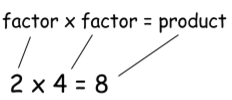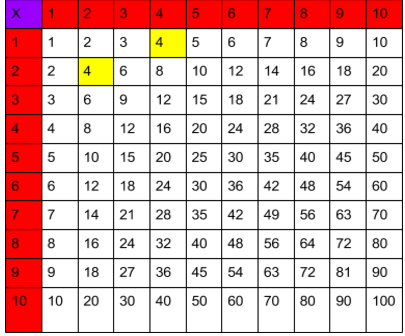# What is a Factor? - Lesson for Kids

Instructor: Dina Albert
When you use multiplication to find a total amount, you are working with factors. In this lesson, we will define what a factor is and explore real-world situations in which you work with factors to solve problems.

## What Is a Factor?

A factor is a number that you multiply with another number to get a product (the solution to a multiplication problem). Think of a multiplication problem as factors being multiplied to find the product. For instance, 2 and 4 are factors of 8:A number can have just two factors or many, many factors. But how? Let's explore further.

## Numbers With Only Two Factors

Let's start by looking at numbers that have only two factors--for example, the number 2. How do you make 2 using multiplication? By multiplying 1 x 2 or 2 x 1. We can say that multiplying 2 and 1 is the only way to make a product of 2. And so, we can also say that 2 has only two factors: 1 and 2.

What about the number 3, how many factors does 3 have? Let's restate the question and ask: What numbers can we multiply together to make a product of 3? Well, the only way to make 3 is by multiplying 1 x 3 or 3 x 1. So, we can say that 3 has only two factors: 1 and 3.

One more example of a number with only two factors is 5. Why? Because we can only make 5 by multiplying 1 x 5 and 5 x 1. So, 5 only has two factors: 1 and 5.

Notice that all three of the numbers we discussed above had factors of 1 and itself.

• 5 had factors of itself (5) and 1
• 3 had factors of itself (3) and 1
• 2 had factors of itself (2) and 1

Numbers such as 2, 3, 5--and any other number that only has factors of itself and 1--are called prime numbers.

## Numbers With More Than Two Factors

Now that we have discussed numbers with only two factors, let's take a look at some numbers that have more than two factors. Numbers that have more than two factors are called composite numbers. Since we skipped the number 4 in our last examples, that's the number we'll start with. This time we will use a multiplication chart to find all the ways we can make 4.To unlock this lesson you must be a Study.com Member.

### Register to view this lesson

Are you a student or a teacher?

#### See for yourself why 30 million people use Study.com

##### Become a Study.com member and start learning now.
Back
What teachers are saying about Study.com

### Earning College Credit

Did you know… We have over 200 college courses that prepare you to earn credit by exam that is accepted by over 1,500 colleges and universities. You can test out of the first two years of college and save thousands off your degree. Anyone can earn credit-by-exam regardless of age or education level.